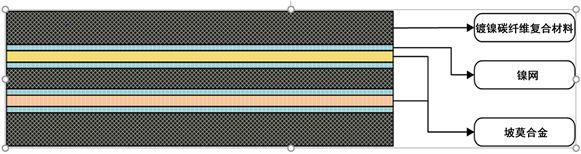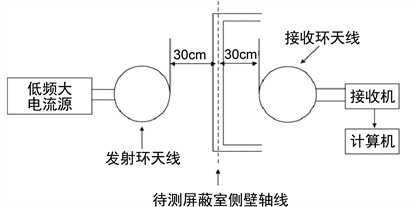# 轨道车辆用低频防护电磁防护碳纤维复合材料研制Development of Carbon Fiber Composite Material for Electromagnetic Protection of Low Frequency Protective Parts of Light-weight Railway Vehicles

• 全文下载: PDF(931KB)    PP.1092-1097   DOI: 10.12677/MS.2019.912134
• 下载量: 94  浏览量: 144

In this paper, according to low-frequency protection design requirements of the railway vehicle electromagnetic sensitive parts, we used the carbon fiber composite material as the main material and combined with the conductive and magnetic interlaminar electromagnetic reinforcement material based on the principle of low frequency electromagnetic protection, the electromagnetic protection carbon fiber composite material suitable for the low frequency electromagnetic pro-tection in railway vehicles was prepared by thermal pressure tank forming process. After the test, the material had the obvious weight reduction compared with the silicon steel system protection material, and the maximum shielding effect was 30 db under the dc magnetic field and the low frequency electromagnetic field (5 Hz~20 KHz). This low-frequency electromagnetic protection carbon fiber composite material had the advantages of light weight, high strength, rapid molding, good shielding performance and strong environmental adaptability, and so on. It had high engi-neering value in the field of intelligent transportation.

1. 引言

2. 直流磁场及低频防护原理

2.1. 直流磁场屏蔽

${\varnothing }_{空气}=\frac{F}{{R}_{空气}}=\frac{IN}{l/\left(S\cdot {\mu }_{空气}\right)}$ (1)

${\varnothing }_{屏蔽}=\frac{F}{{R}_{屏蔽}}=\frac{IN}{l/\left(S\cdot {\mu }_{屏蔽}\right)}$ (2)

2.2. 低频电磁屏蔽

3. 低频防护部位用电磁防护碳纤维复合材料设计

$SE=A+R+M$ (3)

$A=0.314t\sqrt{f{\sigma }_{r}{\mu }_{r}}$ (4)

$r\gg \lambda /2\pi$

${R}_{Pw}=168-10\mathrm{lg}\left(f{\mu }_{r}/{\sigma }_{r}\right)$, 平面波 (5)

$r\ll \lambda /2\pi$

${R}_{EF}=354-20\mathrm{lg}\left(0.39r\sqrt{f{\sigma }_{r}{\mu }_{r}}\right)$, 电场 (6)

$r\gg \lambda /2\pi$

${R}_{MF}=14.6+10\text{lg}\left[\frac{{r}^{2}f{\sigma }_{r}}{{\mu }_{r}}\right]$, 磁场 (7)

$M=20\mathrm{lg}\left[1-{\text{e}}^{-2t/\delta }\right]$ (8)

$\delta =\frac{1}{15.156\sqrt{f{\sigma }_{r}{\mu }_{r}}}$ (9)Figure 1. Schematic diagram of composite layer

4. 电磁防护碳纤维复合材料制造工艺Figure 2. Forming process of autoclaveFigure 3. Curing process parameter curve of autoclave

5. 试验验证

5.1. 性能测试Figure 4. Test diagram

5.2. 结果及分析Table 1. Shielding effectiveness test data

6. 结束语

1) 选用碳纤维、镀镍碳纤维增强材料以及坡莫合金的混合铺层和整体成型工艺过程质量可控，产品有较好的表观质量，在满足强度、刚度等力学性能条件下，实现非常显著的减重效果。

2) 本文研究结论对轨道交通轻量化设计中的电磁兼容和减重设计具有指导意义。

  邓小斌, 侯朝桢. 有孔矩形腔屏蔽效能的传输线法分析[J]. 强激光与粒子束, 2004, 16(3): 341-344.  Kim, M.S., Kim, H.K. and Byun, S.W. (2002) PET Fabric/Polyrrole Composite with High Electrical Conductivity for EMI Shielding. Synthetic Metals, 126, 233-239. https://doi.org/10.1016/s0379-6779(01)00562-8  靳武刚, 高建军. 碳纤维复合材料弯曲撑杆成型工艺技术[J]. 电子机械工程, 2003, 19(3): 62-63.  孙凯, 李敏, 顾铁卓, 等. 热压罐零吸胶工艺树脂压力在线测试及其变化规律[J]. 复合材料学报, 2010, 27(4): 94-99.  陈平, 蹇锡高, 陈辉, 等. 碳纤维复合材料发动机壳体用韧性环氧树脂基体的研究[J]. 复合材料学报, 2002, 19(2): 24 -27.  汝强, 胡社军, 胡显奇, 邱秀丽, 盛钢, 王明. 电磁屏蔽理论及屏蔽材料的制备[J]. 包装工程, 2004, 25(5): 21-23.